# Advanced Tutorial Lesson 13: Exploring Digital Filters

 Tutorial Project: Exploring Digital Filters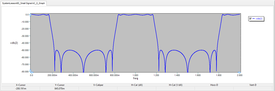Objective: In this project, you will build and test several different types of digital filters. Concepts/Features: Digital Filter Sampling Frequency Delay Element Z-Domain Transfer Function FIR Filter IIR Filter Signal Sampler Random Pulse Generator Minimum Version Required: All versions

## What You Will Learn

In this tutorial you will learn how to use RF.Spice's Generic Digital Filter Block to define finite impulse response (FIR) and infinite impulse response (IIR) filters. You will also use RF.Spice's Signal Sampler Block to create discrete-time versions of continuous-time signals and will learn how to generate noisy waveforms using RF.Spice's Random Pulse Generator.

## A Note on RF.Spice's Generic Digital Filter Block

In RF.Spice A/D the generic digital filter block is defined by its Z-domain transfer function, which is a rational function of the Z-transform variable:

$H(z) = \frac{N(z)}{D(z)} = \frac{ \sum_{m=0}^{M} b_m z^m }{ \sum_{n=0}^{N} a_n z^n }$

with the assumption that N ≥ M.

You need to specify the highest polynomial degree (N) and enter arrays of polynomial coefficients for the numerator and denominator of the transfer function in the property dialog of the generic digital filter block. This part can be accessed from Menu > Parts > Digital Signal Processing Blocks > Generic Digital Filter Block. To open the property dialog of this device, double-click on its symbol and then click the Edit Model... button.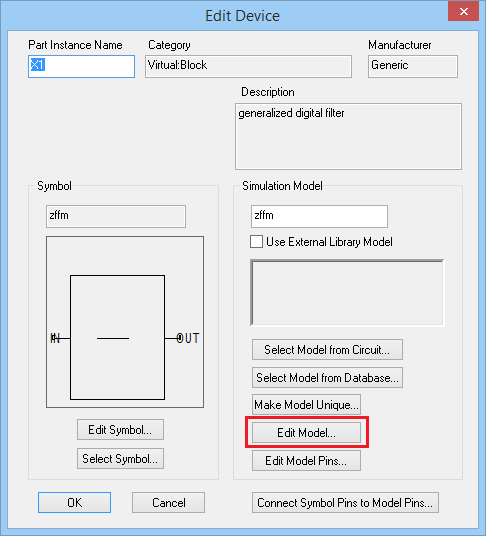The property dialog of the Generic Digital Filter Block: the outer dialog.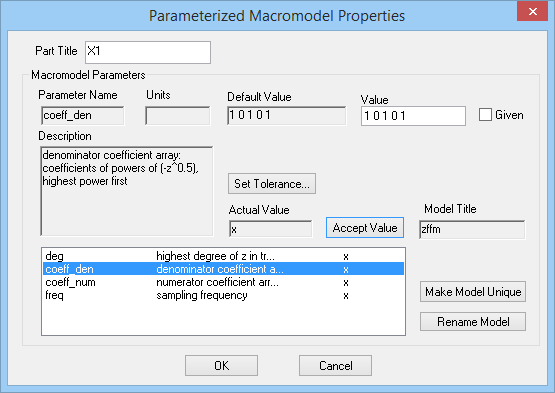The property dialog of the Generic Digital Filter Block: the inner dialog.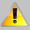For a regular digital filter, you need to insert zero padding among the numerator and denominator polynomial coefficients.

For example, the coefficient array for a polynomial P(z) = z2 + z + 1 must be entered as "1 0 1 0 1". If the degree of the polynomial is N, then you need to enter a total of 2N+1 entries that include N padded zeros. The reason for this is RF.Spice A/D in fact constructs a polynomial in (-z1/2) behind the scene. So in reality, you enter the coefficients for the successive powers of (-z1/2):

$P(z) = z^2 + z + 1 = \sum_{n=0}^{N} a_n z^n = \sum_{k=0}^{2N} a^{\prime}_k (-z^{1/2})^k = 1(-z^{1/2})^4 + 0(-z^{1/2})^3 + 1(-z^{1/2})^2 + 0(-z^{1/2}) + 1$

Note that the term z-1/2 corresponds to a delay equal to half the sampling period.

## Testing a Basic Delay Element

The following is a list of parts needed for this part of the tutorial lesson:

Part Name Part Type Part Value
V1 Finite Sequence Pulse Generator Waveform TBD
R1 Resistor 100
X1 Generic Digital Filter Block Defaults, deg = 1, Numer = "1", Denom = "1 0 0", freq = 1Hz

Place and connect the part as shown in the figure below: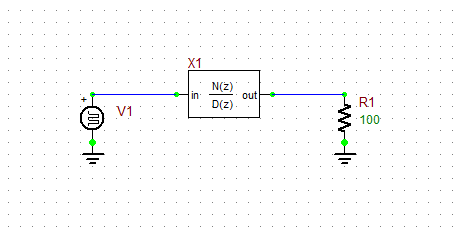A simple circuit to test the generic digital filter block as a basic delay element.

In the first part of this tutorial lesson, you will define a digital filter as a basic delay element. The Z-domain transfer function of this filter is given by:

$H(z) = z^{-1/2} = \frac{1}{z} = \frac {0(-z^{1/2})^2 + 0(-z^{1/2}) + 1}{1(-z^{1/2})^2 + 0(-z^{1/2}) + 0}$

This means that N= 1, M = 0, the coefficients of the denominator must be "1 0 0" and the numerator has only one coefficient "1". You don't have to enter the leading zero coefficients in the numerator.

As the source of your circuit, you are going to define five pulse of period 1s with a duty cycle of 20%. For this purpose, you can use a Finite Sequence Pulse Generator, which can be accessed from Parts > Waveform Generation Blocks > Basic Waveforms >. Set the pulse period to 1s, pulse width to 0.2s, n = 5, and set the rise and fall times both to 5ms.Using pulse waveforms with zero rise or fall times in digital signal processing blocks may cause output overshoots or undershoots.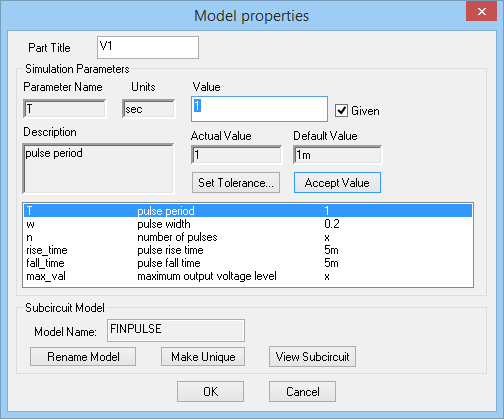The property dialog of the Finite Sequence Pulse Generator.

Run a Transient Test of this circuit with the parameters specified below:

 Start Time 0 8s 10ms 1ms v(1), v(2)

The simulation results are shown in the figure below using the graph window's Separate Plots with Vertical Tiling. You can see that the output waveform is simply the same as the delayed version of the input waveform by one clock cycle (T = 1s).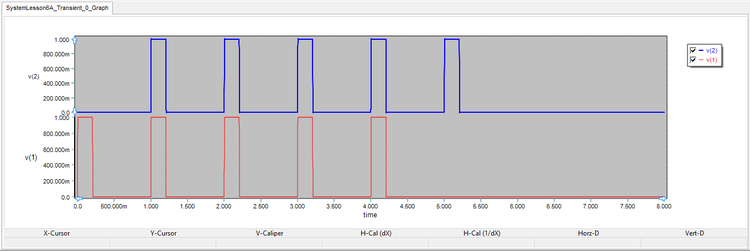The graph of the input and output voltages of the basic delay element.

## Building an FIR Filter: The Moving Average Filter

The following is a list of parts needed for this part of the tutorial lesson:

Part Name Part Type Part Value
V1 Random Pulse Generator Waveform TBD
R1 Resistor 100
X1 Generic Digital Filter Block Defaults, deg = 2, Numer = "1 0 1 0 1", Denom = "3 0 0 0 0", freq = 1Hz

One of the simplest finite impulse response (FIR) digital filters is a moving average filter. A three-point moving average filter is defined by the following transfer function:

$H(z) = \frac{1}{3} \left[ 1 + z^{-1} + z^{-2} \right] = \frac {z^2 + z + 1}{3z^2}$

This filter sums each data sample with delayed versions of the signal by one and two clock cycles, and divides the sum by 3 to get the average. In other words, the relationship between the output and input signal can be expressed as:

$v_o(t) = \frac{1}{3} \left[ v_i(t) + v_i(t-T) +v_i(t-2T) \right]$

where T = 1/fc is the sampling period and fc is the clock frequency. Therefore, M = N = 2 in the case, and the numerator and denominator coefficient arrays are "1 0 1 0 1" and "3 0 0 0 0", respectively.

Place and connect the part as shown in the above figure from the last part. For the input, this time you are going to use a random pulse generator with the parameters specified in the table below. By default, a random pulse generator create pulses with random amplitudes and random widths. The parameter n_rand determines how many possible values of amplitude or pulse width will be considered. In this project, the pulse width will be fixed at w = 0.2s, and only the pulse amplitude will be randomized. That's why you need to set the value of random_amp to 1 and set the value of random_wid to 0.

 period 1s 0.2 1 0 10 5m 5m 1VThe property dialog of the Random Pulse generator.

Run a Transient Test of this circuit with the parameters specified below:

 Start Time 0 100s 10m 1m v(1), v(2)

The simulation results are shown in the figure below for the random pulse input and the filtered output signal:When working with randomized signals, you should expect your results to look different than the graphs shown in these tutorial lessons.The graph of the input and output voltages of the basic delay element.

## Filtering Noisy Signals Using Moving Average Digital Filters

The following is a list of parts needed for this part of the tutorial lesson:

Part Name Part Type Part Value
V1 Sinusoidal Voltage Source Waveform TBD
V2 - V3 Random Pulse Generator Waveform TBD
R1 Resistor 100
A1 Summer Block Defaults
A2 Summer Block Defaults, in_gain = [1 -1], out_gain = 0.15
X1 Signal Sampler Block Defaults, T = 1s, duty_cycle = 0.2, rise_time = 5ms, fall_time = 5ms
X2 Generic Digital Filter Block Defaults, deg = 4, Numer = "1 0 1 0 1 0 1 0 1", Denom = "5 0 0 0 0 0 0 0 0", freq = 1Hz

In this part of the tutorial lesson, you will build a noisy digital signal. To do so, you will take a sinusoidal signal, sample it at a clock rate of 1s using RF.Spice's Signal Sampler Block and add a random pulse waveforms with a peak-to-peak variation of 0.4V. The figure below shows the property dialog of the signal sampler block:The property dialog the Signal Simpler Block.

In order to generate a noisy signal, you can superpose a scaled-down random pulse with your sampled discrete-time signal. However, keep in mind that the output of RF.Spice's random pulse generator fluctutate between 0 and its "max_value". In order to create a noise component fluctuating between positive and negative values, you will use two statistically uncorrelated random pulse generators and sum up their outputs with opposite signs, and scale down (attenuate) the output of the summer. RF.Spice's Summer Block allows you to create a linear combination of the two input signals and also define an output gain as shown in the figure below.

In this circuit you have two different summer blocks A1 and A2 with different properties. For basic summer A2, you will accept all the default parameter values. Since the Summer Block is a native XSPICE process device, by default all of its instances share the same model unless you force a certain part to have a "Unique Model".

For this part of the tutorial lesson, you will define a 5-point moving average filter with the following Z-domain transfer function:

$H(z) = \frac{1}{5} \left[ 1 + z^{-1} + z^{-2} + z^{-3} + z^{-4} \right] = \frac {z^4 + z^3 + z^2 + z + 1}{5z^4}$

In this case, M = N = 4, and the numerator and denominator coefficient arrays are "1 0 1 0 1 0 1 0 1" and "5 0 0 0 0 0 0 0 0", respectively.

Place and connect the part as shown in the above figure from the last part. Define the waveforms of the sinusoidal source V1 and the two random pulse generators V2 and V3 according to the table below:

 Offset Voltage 0 1V 0.02Hz 0 0

 period 1s 0.2 1 0 10 5m 5m 1V

Place and connect all the parts as shown in the figure below: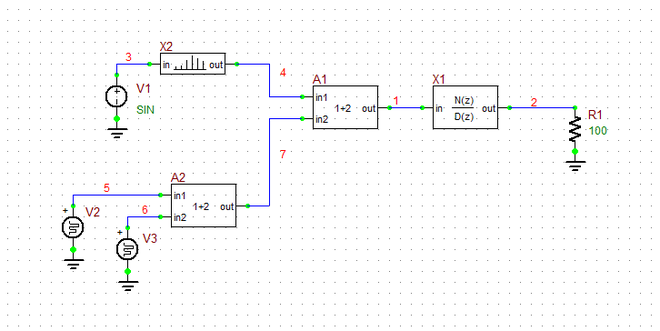The schematic of a noisy discrete-time sinusoidal waveform generator circuit with a moving average digital filter.

Run a Transient Test of this circuit with the parameters specified below:

 Start Time 0 100s 10m 1m v(1), v(2), v(3), v(7)

The simulation results are shown in the figure below. v(7) is the random noise signal with a maximum peak-to-peak amplitude of 0.3V before being superposed with the sampled sinusoid. v(1) is the noisy discrete-time sinusoidal signal. From the plot of the output signal v(2) in the figure, you can see that the digital filter cleans up and smoothes out the noisy input signal.The graph of the input and output signals with with a maximum peak-to-peak noise fluctuation of 0.3V and n_rand = 10.

Next, open the property dialog of the two random pulse generators V2 and V3 and set their number of random amplitude levels to n_rand = 20. Also, in the property dialog of the summer block A1, set the value of out_gain equal to 0.4. This will increase the peak-to-peark noise amplitude to 0.8V superimposed on a sinusoid with a peak-to-peak amplitude of 2V. Run another transient test of your system an plots the signal graphs as shown in the figure below. You can clearly see that the input signal to the digital filter has now become much noisier, and the filter is still doing a quite decent job of removing the noise.The graph of the input and output signals with with a maximum peak-to-peak noise fluctuation of 0.8V and n_rand = 20.

## Building & Testing a Recursive Digital Filter

The following is a list of parts needed for this part of the tutorial lesson:

Part Name Part Type Part Value
V1 AC Voltage Source 1V
R1 Resistor 100
X1 Generic Digital Filter Block Defaults, deg = 1, Numer = "1", Denom = "1 0 0", freq = 1Hz

Recursive digital filters, also know as Infinite Impulse Response (IIR) filters, have many applications including the digital or discrete-time counterparts of classic continuous-time filters. The Z-domain transfer function of a fifth-order elliptic lowpass digital filter is given by:

$H(z) = \frac{ 0.01943z^5 + 0.02111z^4 + 0.03771z^3 + 0.03771z^2 + 0.02111z + 0.01943 }{ z^5 - 2.7580z^4 + 4.0110z^3 - 3.3711z^2 + 1.6542z - 0.3796 }$

In this case, M = N = 5, and the eleven numerator and denominator array coefficients are given by:

N(z): 0.01943 0 0.02111 0 0.03771 0 0.03771 0 0.02111 0 0.01943

D(z): 1 0 -2.7580 0 4.0110 0 -3.3711 0 1.6542 0 -0.3796

Place and connect the part as shown in the figure below:The schematic of the elliptic digital filter.

You are primarily interested in the frequency response of this digital filter. Therefore, run an AC frequency sweep of your filter with the following parameters:

 Start Frequency 1mHz 2Hz 0.5mHz Linear VDB(2)

The frequency response of the digital filter is shown in the figure below, where you can see a lowpass behavior. Note that the frequency response of digital filters is periodic with a period equal to the sampling period, which is 1Hz in this case.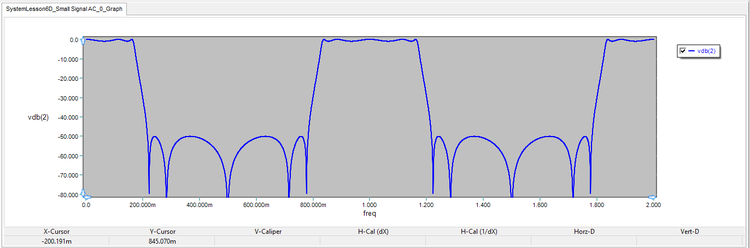The frequency response of the second-order Butterworth loswpass filter.

## Examining the Transient Response of an IIR Digital Filter

For the last part of this tutorial lesson, you are going to use the circuit of the previous part. But you will replace the AC voltage source with a Finite Sequence Pulse Generator identical to the one you used in the first part of the lesson. The following are the source's specifications:

 pulse period 1s 0.2s 5 5m 5m 1V

Run a Transient Test of this circuit with the parameters specified below:

 Start Time 0 20s 10ms 1ms v(1), v(2)

The simulation results are shown in the figure below. As you can see from the figure, even though your input pulse sequence has a finite duration or 5s, its output has an infinite duration. But is decays very fast.The graph of the input and output voltage signals of the elliptic IIR digital filter.